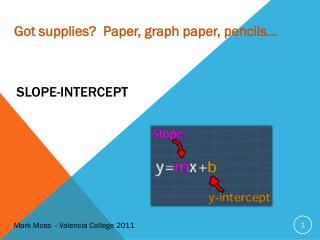DownloadDownload PresentationSlope -intercept

# Slope -intercept

Download Presentation## Slope -intercept

- - - - - - - - - - - - - - - - - - - - - - - - - - - E N D - - - - - - - - - - - - - - - - - - - - - - - - - - -
##### Presentation Transcript

1. Got supplies? Paper, graph paper, pencils… Slope-intercept Mark Moss - Valencia College 2011

2. OBJECTIVES: Where we are going to: Define slope-intercept form. Rewrite standard and slope-intercept forms. Graph lines from various data. Slope-intercept rate of change plus y-intercept Mark Moss – Valencia College 2011

3. What is the Slope-intercept form?

4. y 3x + 2 Graphing the Slope-intercept form x

5. y -2 Compare & contrast 3x + 2 x

6. y 1/4x + 5 Visualizing the Slope-intercept form x 1/4x - 5

7. y 1/4x + 5 Visualizing the Slope-intercept form x -1/4x - 5

8. y 1/4x + 5 Visualizing the Slope-intercept form x -4x - 5

9. 5x + 10y = 200 5x + 10y = 200 Rewriting standard form to slope-intercept form? 5x + 10y = 200 5(0) + 10y = 200 10y = 200 y= 20 (0,20) 5x + 10y = 200 5x + 10(0) = 200 5x = 200 X= 40 (40,0) 5x + 10y = 200 ( add -5x) -5x+5x+10y = -5x+200 10y = -5x +200 (multiply 1/10) ( y = x + 20 m = 0 – 20 / 40 – 0 = -1/2

10. y How do we graph this? Let’s put it all together x

11. y What is the equation of this line? Let’s put it all together x

12. y How do you graph this? Let’s put it all together x

13. Can we put these into standard form? 2 Let’s put it all together

14. Can we put these into slope-intercept form? 2 Let’s put it all together

15. OBJECTIVES You will be able to define slope-intercept. Translate between standard and slope-intercept forms. Graph lines given combinations of various data. Slope-intercept If you can read this you made it to the top Mark Moss – Valencia College 2011